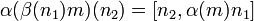# Compatible pair of actions of Lie rings

## Definition

Suppose$M$ and$N$ are Lie rings. Suppose$\alpha:M \to \operatorname{Der}(N)$ and$\beta:N \to \operatorname{Der}(M)$ are homomorphisms of Lie rings, where$\operatorname{Der}(M)$ and$\operatorname{Der}(N)$ denote the Lie ring of derivations of$M$ and of$N$ respectively. We say that$\alpha,\beta$ form a compatible pair of actions if the following two conditions hold:

•$\alpha(\beta(n_1)m)(n_2) = [n_2,\alpha(m)n_1]$ for all$m \in M$ and for all$n_1,n_2 \in N$ (possibly equal).
•$\beta(\alpha(m_1)n)(m_2) = [m_2,\beta(n)m_1]$ for all$m_1,m_2 \in M$ (possibly equal) and for all$n \in N$.

The above expressions are easier to write down if we use$\cdot$ to denote the actions. In this case, the above become:

•$(n_1 \cdot m) \cdot n_2 = [n_2,m \cdot n_1]$ for all$m \in M$ and for all$n_1,n_2 \in N$ (possibly equal).
•$(m_1 \cdot n) \cdot m_2 = [m_2, n \cdot m_1]$ for all$m_1,m_2 \in M$ (possibly equal) and for all$n \in N$.

### With additional Lie algebra structure

If$M$ and$N$ both have the structure of Lie algebras over a common base ring$R$, and the maps$\alpha,\beta$ are actions compatible with the$R$-module structure, then the above definition defines the notion of compatible pair of actions of Lie algebras.

## Particular cases

• Trivial pair of Lie ring actions is compatible: If both the actions are trivial, i.e., both the homomorphisms$\alpha,\beta$ are trivial maps, then they form a compatible pair.
• If$M,N$ are both subrings of some Lie ring$Q$ that normalize each other (i.e., each is contained in the normalizer of the other), and$\alpha,\beta$ are the adjoint actions of the Lie rings on each other (i.e., the restrictions of the inner derivations induced by the element), then they form a compatible pair. Note that in this case, all the actions are just restrictions of inner derivations and checking the conditions simply amounts to checking the equality of two iterated Lie bracket expressions.